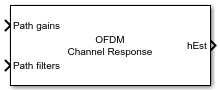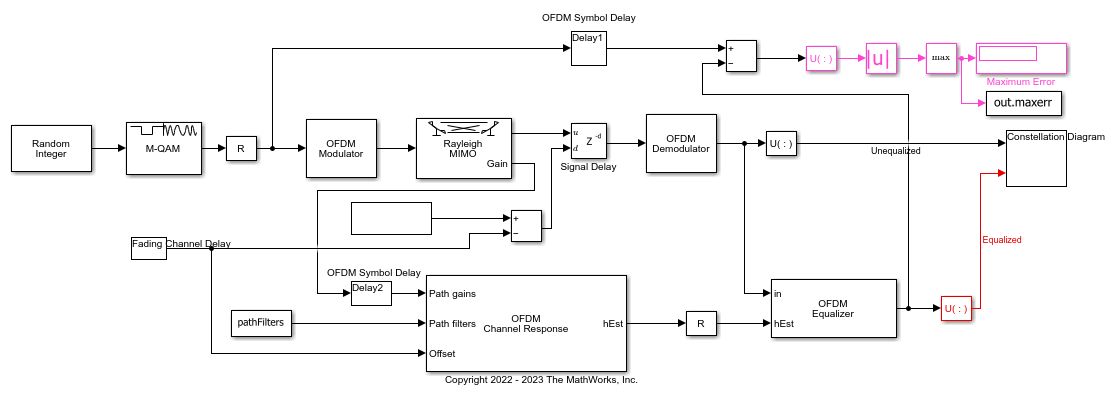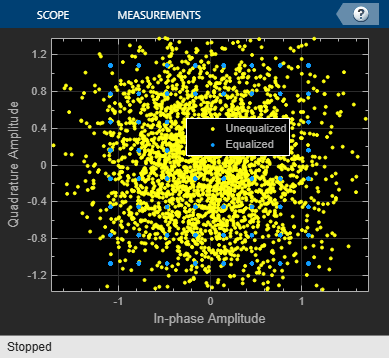# OFDM Channel Response

Calculate OFDM channel response

Since R2023a

•Libraries:
Communications Toolbox / Channels

## Description

The OFDM Channel Response block calculates the OFDM channel response in the frequency domain by using perfect information from the channel.

This icon shows the block with all ports enabled.## Examples

expand all

Apply equalization to an OFDM-modulated QAM signal that has been filtered through a Rayleigh MIMO channel.

The `cm_ofdm_equalization` model initializes the simulation variable in the `InitFun` callback function. For more information, see Model Callbacks (Simulink).The model generates random integer data, applies 64-QAM, and then applies OFDM to the QAM-modulated signal. The OFDM-modulated signal gets filtered through a MIMO Rayleigh fading channel. The model adds a signal delay, and then OFDM-demodulates the signal. In a parallel path, an OFDM Channel Response block computes the perfect OFDM channel response and an OFDM Equalizer block equalizes the received signal. A constellation diagram displays the unequalized and equalized signals. Compare the transmitted QAM signal and the equalized signal on the receive side to compute the error.

`The maximum computed error is 0.000558.`## Ports

### Input

expand all

Channel path gains, specified as an NS-by-NP-by-T-by-NR array of values.

• NS is the number of samples.

• NP is the number of paths.

• NT is the number of transmit antennas.

• NR is the number of receive antennas.

The channel path gains follow the definition of channel path gains as calculated and output by the fading channel System objects. For example, see MIMO Fading Channel or SISO Fading Channel.

Data Types: `single` | `double`
Complex Number Support: Yes

Channel path filter coefficients, specified as an NP-by-NH matrix of values.

• NP is the number of paths.

• NH is the number of impulse response samples.

The channel path filter coefficient matrix is used to convert path gains to channel filter tap gains for each sample and each pair of transmit and receive antennas. The channel path filter coefficients follow the definition of channel path filter coefficients as calculated by the `info` object function of the fading channel System objects.

The channel path gains follow the definition of channel path gains as calculated and output by the fading channel System objects. For example, see MIMO Fading Channel or SISO Fading Channel.

Data Types: `single` | `double`
Complex Number Support: Yes

Timing offset in samples, specified as a nonnegative, integer scalar.

#### Dependencies

Data Types: `double` | `single`

### Output

expand all

Frequency response, returned as an NSC-by-NSym-by-NT-by-NR array.

• NSC is the number of OFDM subcarriers and equals the length of Active subcarrier indices.

• NSym is the number of OFDM symbols.

• NT is the number of transmit antennas.

• NR is the number of receive antennas.

## Parameters

expand all

To edit block parameters interactively, use the Property Inspector. From the Simulink® Toolstrip, on the Simulation tab, in the Prepare gallery, select .

FFT length, specified as a positive, integer scalar. The FFT length must align with that of the OFDM-modulated signal.

Cyclic prefix length of one OFDM symbol, specified as a nonnegative, integer scalar with a value less than FFT length.

Number of active subcarriers, specified as a positive, integer scalar or column vector of values in the range [`1`, FFT length].

Select this parameter to add an input port to input timing offset.

• `on` — Adds the Offset input port to specify the timing offset for channel response estimation.

• `off` — The block uses the Path gains and Path filters inputs to estimate the timing offset for OFDM channel response estimation.

Type of simulation to run, specified as ```Interpreted execution``` or `Code generation`.

• `Interpreted execution` — Simulate the model by using the MATLAB® interpreter. This option requires less startup time, but the speed of subsequent simulations is slower than with the ```Code generation``` option. In this mode, you can debug the source code of the block.

• `Code generation` — Simulate the model by using generated C code. The first time you run a simulation, Simulink generates C code for the block. The model reuses the C code for subsequent simulations unless the model changes. This option requires additional startup time, but the speed of the subsequent simulations is faster than with the `Interpreted execution` option.

## Block Characteristics

 Data Types `double` | `single` Multidimensional Signals `yes` Variable-Size Signals `yes`

## Version History

Introduced in R2023a